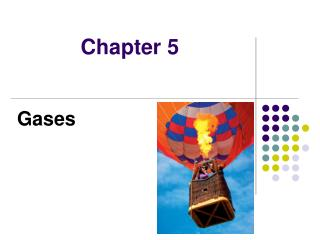DownloadDownload PresentationChapter 5

# Chapter 5

Download Presentation## Chapter 5

- - - - - - - - - - - - - - - - - - - - - - - - - - - E N D - - - - - - - - - - - - - - - - - - - - - - - - - - -
##### Presentation Transcript

1. Chapter 5 Gases

2. Early Experiments • Torricelli performed experiments that showed that air in the atmosphere exert pressure. • Torricelli designed the first barometer.

3. A torricellian barometer

4. Simple manometer

5. Unit of Pressure 1 standard atmosphere =1 atm =760 mm Hg =760 torr =101325 Pa

6. Boyle’s Experiment

7. A gas that obeys Boyle’s law is called an ideal gas Boyle’s Law : PV=k

8. Charles’s Law: V=bT • The volume of a gas at constant pressure increases linearly with the temperature of the gas.

9. Plot V vs. T using kelvin scale

10. Avogadro’s Law : V=an • A gas at constant temperature and pressure the volume is directly proportional to the number of moles of gas.

11. Ideal Gas Law Equation of state for a gas • PV=nRT • P: atm • V: Liter • n: moles • R: 0.082 L atm K-1 • T: K

12. Laws for Gas experiments • Boyle’s Law : PV=k • Charles’s Law : V=bT • Avogadro’s Law : V=an • The ideal Gas Law : PV=nRT (so called equation of state for idea gas)

13. Ideal Gas • The volume of the individual particles can be assumed to be negligible. • The particles are assumed to exert no force on each other. • It expresses behavior that real gases approach at low pressure and high temperature.

14. Gas Stoichiometry Standard Temperature and Pressure (STP) • T=0oC • P=1 atm • V=22.4 L Natural Temperature and Pressure (NTP) • T=25oC • P=1 atm • V=24.5L

15. Plot of PV versus P for 1 mol of ammonia.

16. 2NaN3(s)→2Na(s)+3N2(g)

17. Dalton’s Law of Partial Pressures

18. Mole Fraction and Partial Pressure

19. The Model of Ideal Gas in Kinetic-Molecular Theory • The volume of the individual particles can be assumed to be negligible. • The particles are assumed to exert no force on each other. • The particles are in constant motion. • The average kinetic energy of a collection of gas particles is assumed to be directly proportional to the Kelvin temperature of the gas.

20. The velocity u can be broken down into three perpendicular components, ux, uy, and u2.

21. Pressure of an Ideal Gas Let the container be a rectangular box with sides of length Lx, Ly and Lz. Let v be the velocity of a given molecule.

22. In the xy plane,vx2 + vy2 = vyx2 by the Pythagorean theorem.

23. A molecule collide with wall W where W is parallel to the xz plane. Let i have the velocity components vx, vy, vz

24. The integration can be extended over the whole time interval t1 to t2. 速度平方之平均值

25. The translational energy Etr= 1/2mv2 single particle

26. Temperature dependence with translation kinetic energy one mole of particles single particle

27. Distribution of Molecular Speeds in an Ideal Gas Root mean square speed is assumed that all molecules move at the same speed. The motions of gas molecules should have distribution of molecular speeds in equilibrium.

28. 指數函數→機率

29. Plot of O2 molecules

30. Plot of N2 molecules

31. Application of The Maxwell Distribution

32. Application of The Maxwell Distribution

33. Velocity distribution for nitrogen

34. Collisions with a Wall vydt ly dNw:粒子撞擊牆壁的數目

35. H2 at 25oC and 1atm 分子量單位:Kg

36. Definition of Pressure • The pressure of a gas results from collisions between the gas particles and the walls of the container. • Each time a gas particle hits the wall, it exerts a force on the wall. • An increase in the number of gas particles in the container increases the frequency of collisions with the walls and therefore the pressure of the gas.

37. The effusion of a gas into an evacuated chamber.

38. Suppose there is a tiny hole of area A in the wall and that outside the container is a vacuum. Escape of a gas through a tiny hole is called effusion. collisions × area

39. Diffusion Relative diffusion rates of NH3 and HCl molecules

40. Molecules Collisions and Mean Free Path • Intermolecular collisions are important in reaction kinetics. • Assume a molecule as a hard sphere. • No intermolecular forces exist except at the moment of collision. zAA: the number of collisions per unit time that one particular A molecule makes with other A molecule [collisions s-1]

41. Cylinder swept by gas particles

42. Calculate zAA and zAB (rA+rB=d)

43. A B